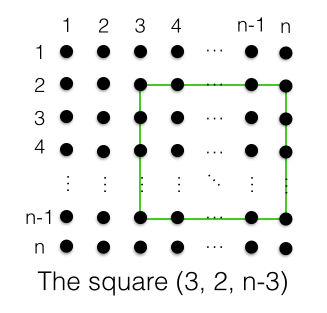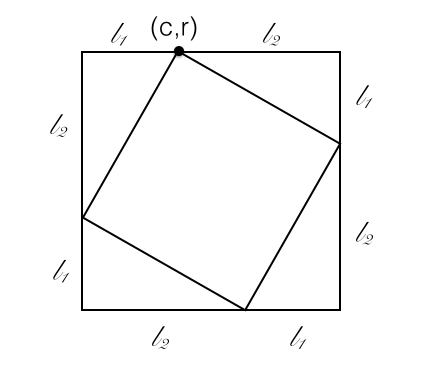## Squares in a Lattice Grid

2017 Feb 24

How many possible squares can be constructed by connecting some $4$ points of an $n$ x $n$ grid of lattice points? What about in a rectangular $m$ x $n$ grid of lattice points?

First one must notice that there are essentially two types of squares: normal squares with vertical and horizontal sides and diagonal squares with sloped sides. The formula giving the total number of possible squares will be the sum of the formulas for the number of normal squares and for the number of diagonal squares.

Suppose we have an $n$ x $n$ grid of lattice points. Let us denote a normal square on such a grid by an ordered triple $(c_0, r_0, l_0)$ where $c_0$ is the column of the square's leftmost side, $r_0$ is the row of the square's topmost side, and $l_0$ is the side length of the square in units.If a square with such an ordered triple sits in an $n$ x $n$ grid of lattice points, none of $c_0$, $r_0$, $c_0+l$, and $r_0+l$ can exceed $n-1$. This means that for any value of $l$, there are $n-l$ possible values for both $c_0$ and $r_0$, meaning that there are a total of $(n-l)^2$ possible values for $c_0$ and $r_0$ together for each value of $l$. Since $l$ can take on a minimum value of $1$ and a maximum value of $n-1$, the total number of normal squares is

Which is equal to

So now we have the total number of normal squares and we need to find the total number of diagonal squares.

Now let us denote a lattice point in the cth column and the rth row as $(c,r)$. Let the values $l_1$ and $l_2$ be defined as shown in the picture for any diagonal square:Such a diagonal square with side length $\sqrt{{l_1}^2+{l_2}^2}$ fits perfectly inside of a square with side length $l_1+l_2$, so the number of possible diagonal squares with some given value of $l_1+l_2$ is equal to the number of normal squares with side length $l_1+l_2$. Let $l_s=l_1+l_2$. Then we know from our analysis of normal squares that the number of normal squares with side length $l_s$ is $(n-l_s)^2$. Additionally, for any possible value of $l_s$, there are $l_s-1$ possible values of $l_1$ and $l_2$ so that $l_1+l_2=l_s$ and both $l_1$ and $l_2$ are positive integers. This means that the total number of diagonal squares for any value of $l_s$ is

In our grid of lattice points, the greatest possible value of $l_s$ is $n-1$, so the total number of diagonal squares is the sum of the number of possible squares with values of $l_s$ from $2$ to $n-1$ since the minimum value of $l_1+l_2$ is $2$ and the maximum is $n-1$, giving us

which we can convert to a closed-form formula (thanks, Wolfram Alpha!) :

The total number of squares is the sum of our two formulas:

Giving us a simple formula for the total number of normal and diagonal squares in an $n$ x $n$ grid of lattice points.

Using a similar method, we can derive the formula for the total number of squares in a rectangular $m$ x $n$ grid of lattice points, where $m \ge n$: